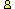CTK Exchange Front Page Movie shortcuts Personal info Awards Terms of use Privacy Policy Cut The Knot! MSET99 Talk Games & Puzzles Arithmetic/Algebra Geometry Probability Eye Opener Analog Gadgets Inventor's Paradox Did you know?... Proofs Math as Language Things Impossible My Logo Math Poll Other Math sit's Guest book News sit's Recommend this siteCTK Exchange

 Subject: "Ceva Theorem By Signed Circumradii" Previous Topic | Next Topic
 ConferencesThe CTK ExchangeCollege mathTopic #687Printer-friendly copyEmail this topic to a friend Reading Topic #687
Bui Quang Tuan
Member since Jun-23-07
Aug-01-08, 09:17 AM (EST)"Ceva Theorem By Signed Circumradii"

 Dear All My Fiends,Given triangle ABC with side lengths a, b, c and A', B', C' are points on sidelines BC, CA, AB respectively. (O) is circumcircle of ABC with radius R. A'', B'', C'' are intesections of AA', BB', CC' with (O) respectively (other than A, B, C).We define six signed circumradii of six triangles:r(BA') = circumradius of triangle BA''A'r(CA') = circumradius of triangle CA''A'r(CB') = circumradius of triangle CB''B'r(AB') = circumradius of triangle AB''B'r(AC') = circumradius of triangle AC''C'r(BC') = circumradius of triangle BC''C'If vertices of the triangle follow the directions of vertices of ABC then its circumradius is positive, otherwise its circumradius is negative. Similarly we define signed segments on the sidelines of ABC. Of course r(XY) = - r(YX). From now, all radii and segments are signed.Angle(AA''B) = Angle(ACB) or = 180 - Angle(ACB)therefore (by sinus theorem):r(BA')/R = BA'/AB = BA'/c and r(BA') = (BA'/c)*RSimilarly: r(CA') = (CA'/b)*R or r(A'C) = (A'C/b)*RFrom these: r(BA')/r(A'C) = (BA'/A'C)*(b/c)Similarly we can show:r(BA')/r(A'C) = (BA'/A'C)*(b/c)r(CB')/r(B'A) = (CB'/B'A)*(c/a)r(AC')/r(C'B) = (AC'/C'B)*(a/b)Multiply these three expressions we have:r(BA')/r(A'C)*r(CB')/r(B'A)*r(AC')/r(C'B) =(BA'/A'C)*(CB'/B'A)*(AC'/C'B)The right part is part of Ceva theorem so we can say:r(BA')/r(A'C)*r(CB')/r(B'A)*r(AC')/r(C'B) = 1 if and only if AA', BB', CC' are concurrent.Because this fact related Ceva theorem so I am not sure that this fact is well known or not. Please kindly give me your reference if you know about it'somewhere!Thank you and best regards,Bui Quang Tuan

Alert | IP Printer-friendly page | Reply | Reply With Quote | Top
alexbCharter Member
2249 posts
Aug-02-08, 08:05 PM (EST)1. "RE: Ceva Theorem By Signed Circumradii"
In response to message #0

 For whatever it is worth: this is a curiosity I have not seen before.

Alert | IP Printer-friendly page | Reply | Reply With Quote | Top

 Conferences | Forums | Topics | Previous Topic | Next Topic
 Select another forum or conference Lobby The CTK Exchange (Conference)   |--Early math (Public)   |--Middle school (Public)   |--High school (Public)   |--College math (Public)   |--This and that (Public)   |--Guest book (Protected)   |--Thoughts and Suggestions (Public) Educational Press (Conference)   |--No Child Left Behind (Public)   |--Math Wars (Public)   |--Mathematics and general education (Public)You may be curious to have a look at the old CTK Exchange archive.
Please do not post there.